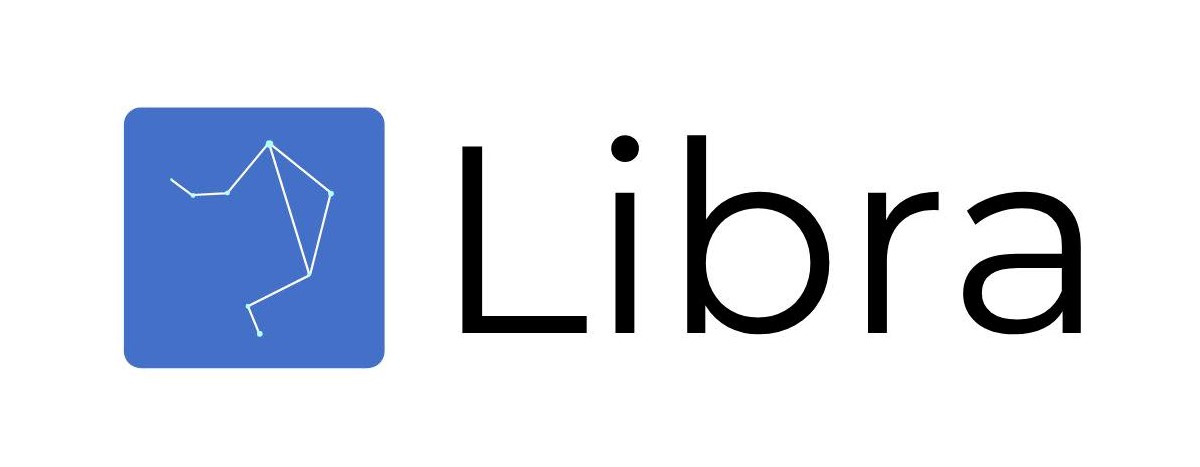# dashboard()

Launches a fully functional UI to perform data analysis and dimensionality reduction live!

`new_client = client('path_to_dataset').dashboard()`

# analyze()

Generates in-depth statistics about your dataset. Creates confusion matrix and ROC Curve for all classification problems. Also calculates recall, precision, f1, and f2 score. For regression problems, this function will calculate MSE, MAE, and other important statistics.

 Arguments model=latest_model The model that you want to analyze. If you want to call on the latest model, this parameter does not need to be specified. save_model=False Whether you want to save your model. Will generate a .json and a .h5 file. save=True Whether you want to save the plots that are generated as .png files.
```new_client = client('path_to_dataset')
new_client.neural_network_query('Please estimate the number of households.')
new_client.analyze()```

# info()

Returns all the keys, representing each category of data generated for the dataset.

 Arguments model=latest_model The model that you want to analyze. If you want to call on the latest model, this parameter does not need to be specified.
```new_client = client('path_to_dataset')
new_client.neural_network_query('Please estimate the number of households.')
new_client.info() #access plots```

# plots()

Displays all of the plots generated for the model it is called on.

 Arguments model=latest_model The model that you want to analyze. If you want to call on the latest model, this parameter does not need to be specified. plot=None The plot that you want to display. If not specified, all plots will be displayed. save=False Whether you want to save the plots that are generated as .png files.
```new_client = client('path_to_dataset')
new_client.neural_network_query('Please estimate the number of households.')
new_client.plots() #access plots```

# vocab()

Returns the vocabulary used for the query. Can only be used for document summarization and image caption.

 Arguments data The data that you want to predict for this model. model=latest_model The model that you want to analyze. If you want to call on the latest model, this parameter does not need to be specified.
```new_client = client('path_to_dataset')
new_client.summarization_query('csv_file')
new_client.vocab() #access vocabulary```

# predict()

Automatically fits a neural network to your dataset. Target detection, preprocessing, and scoring are done by default.

 Arguments model=latest_model The model that you want to analyze. If you want to call on the latest model, this parameter does not need to be specified.
```new_client = client('path_to_dataset')
new_client.neural_network_query('Please estimate the number of households.')
new_client.predict(new_data, model='regression_ANN')```

# model()

Returns the entire dictionary that a specific model.

 Arguments model=latest_model The model that you want to analyze. If you want to call on the latest model, this parameter does not need to be specified.
```new_client = client('path_to_dataset')
new_client.neural_network_query('Please estimate the number of households.')
new_client.model()['plots'] #access plots```